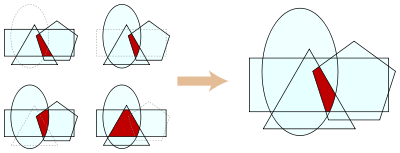# Helly's theoremHelly's theorem for the Euclidean plane: if a family of convex sets has a nonempty intersection for every triple of sets, then the whole family has a nonempty intersection.

Helly's theorem is a basic result in discrete geometry on the intersection of convex sets. It was discovered by Eduard Helly in 1913, but not published by him until 1923, by which time alternative proofs by Radon (1921) and König (1922) had already appeared. Helly's theorem gave rise to the notion of a Helly family.

## Statement

Let X1, ..., Xn be a finite collection of convex subsets of Rd, with n > d. If the intersection of every d + 1 of these sets is nonempty, then the whole collection has a nonempty intersection; that is,For infinite collections one has to assume compactness:

Let {Xα} be a collection of compact convex subsets of Rd, such that every subcollection of cardinality at most d + 1 has nonempty intersection, then the whole collection has nonempty intersection.

## Proof

We prove the finite version, using Radon's theorem as in the proof by Radon (1921). The infinite version then follows by the finite intersection property characterization of compactness: a collection of closed subsets of a compact space has a non-empty intersection if and only if every finite subcollection has a non-empty intersection (once you fix a single set, the intersection of all others with it are closed subsets of a fixed compact space).

The proof is by induction:

Base case: Let n = d + 2. By our assumptions, for every j = 1, ..., n there is a point xj that is in the common intersection of all Xi with the possible exception of Xj. Now we apply Radon's theorem to the set A = {x1, ..., xn}, which furnishes us with disjoint subsets A1, A2 of A such that the convex hull of A1 intersects the convex hull of A2. Suppose that p is a point in the intersection of these two convex hulls. We claim thatIndeed, consider any j ∈ {1, ..., n}. We shall prove that pXj. Note that the only element of A that may not be in Xj is xj. If xjA1, then xjA2, and therefore XjA2. Since Xj is convex, it then also contains the convex hull of A2 and therefore also pXj. Likewise, if xjA1, then XjA1, and by the same reasoning pXj. Since p is in every Xj, it must also be in the intersection.

Above, we have assumed that the points x1, ..., xn are all distinct. If this is not the case, say xi = xk for some ik, then xi is in every one of the sets Xj, and again we conclude that the intersection is nonempty. This completes the proof in the case n = d + 2.

Inductive Step: Suppose n > d + 2 and that the statement is true for n−1. The argument above shows that any subcollection of d + 2 sets will have nonempty intersection. We may then consider the collection where we replace the two sets Xn−1 and Xn with the single set Xn−1Xn. In this new collection, every subcollection of d + 1 sets will have nonempty intersection. The inductive hypothesis therefore applies, and shows that this new collection has nonempty intersection. This implies the same for the original collection, and completes the proof.